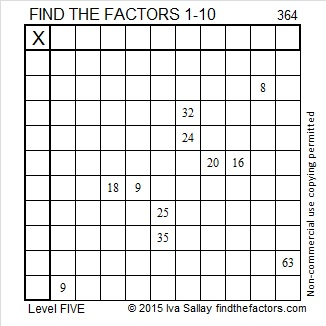# 364 and Level 5

364 is an easy tetrahedral number to remember because it is one less than the number of days in a year. It is the ridiculous sum total number of all the birds, rings, maids, dancers, and musicians given over the twelve days of Christmas.

One of 364’s factor pairs is also easy to remember: 7 × 52 = 364. There are 7 days in a week and 52 weeks in a year . . . or rather in a year minus one day.

364 is in this cool pattern:The factoring information for 364 is below the puzzle.Print the puzzles or type the factors on this excel file: 10 Factors 2015-01-19

• 364 is a composite number.
• Prime factorization: 364 = 2 x 2 x 7 x 13, which can be written 364 = (2^2) x 7 x 13
• The exponents in the prime factorization are 2, 1, and 1. Adding one to each and multiplying we get (2 + 1)(1 + 1)(1 + 1) = 3 x 2 x 2 = 12. Therefore 364 has exactly 12 factors.
• Factors of 364: 1, 2, 4, 7, 13, 14, 26, 28, 52, 91, 182, 364
• Factor pairs: 364 = 1 x 364, 2 x 182, 4 x 91, 7 x 52, 13 x 28, or 14 x 26
• Taking the factor pair with the largest square number factor, we get √364 = (√4)(√91) = 2√91 ≈ 19.079This site uses Akismet to reduce spam. Learn how your comment data is processed.